Home MATHEMATICS TOPIC 2: ALGEBRA ~ MATHEMATICS FORM 2

# TOPIC 2: ALGEBRA ~ MATHEMATICS FORM 2

1548
4### TOPIC 2: ALGEBRA ~ MATHEMATICS FORM 2

Once we learn some of the ‘tricks’ it becomes a fun challenge to work with our skills in solving each puzzle. So, Algebra is all about solving puzzles. In this
chapter we are going to learn some of the skills that help in solving mathematics puzzles.

The Binary Operations
Describe the binary operations
When two numbers are combined according to the instructions given and produce one number we say that they are binary operations. For example, when we add 4 to 6 we get 10, or when we multiply 4 by 3 we get 12. We see that addition of two numbers lead to one number and multiplication of two numbers produce one number. This is binary operation. The instructions can be given either by symbols like X,*,∇and so on or by
words.
Performing Binary Operations
Perform binary operations
Example 1
Evaluate: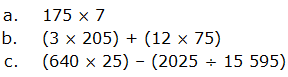solution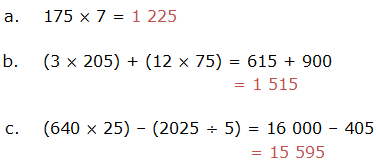Example 2
Find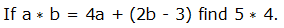solution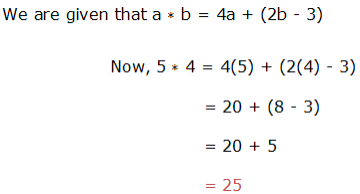Example 3
Solve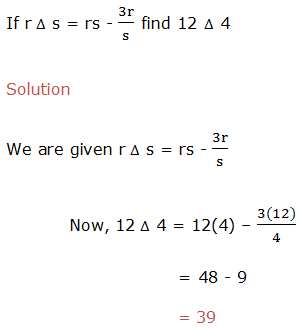Example 4
evaluate,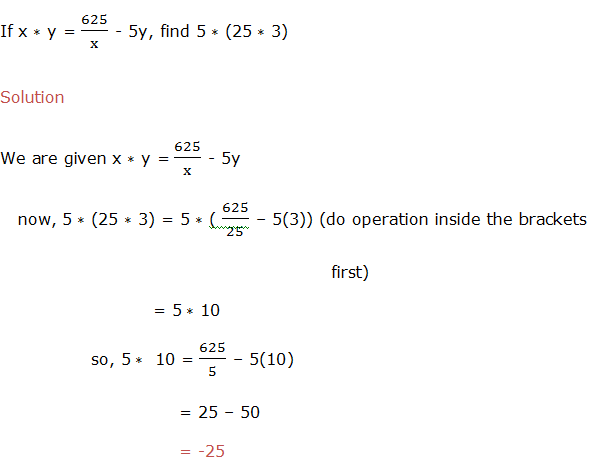Example 5
Calculate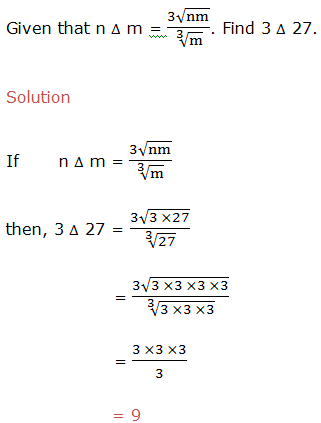Linear Expressions
Factorize linear expressions
The
operation of resolving a quantity into factors, when we expand
expressions, is done by removing the brackets. The reverse operation is
Factorizing and it is done by adding brackets.
Example 11
Factorize the expression 5a+5b.
Solution
In
factorization of 5a+5b, we have to find out a common thing in both
terms. We can see that the expression 5a+5b, have got common coefficient
in both terms, that is 5. So factoring it out we get 5(a+b).
Example 12
Factorize 18xyz-24xwz
Solution
Factorizing
18xyz-24xwz, we have to find out highest common factor of both terms.
Then factor it out, the answer will be 9xz(2y-3w).
When
we write the quadratic expression as a product of two factors we say
that we have factorized the expression. We are going to learn two
methods used to factorize quadratic expressions. These methods are
factorization by Splitting the middle term and factorization by
Inspection.
Factorization by splitting the middle term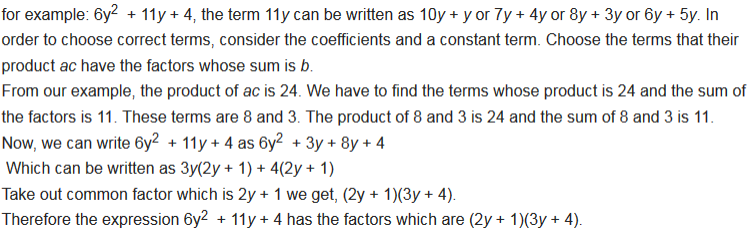Example 13
factorize 3x2 – 2x – 8 by splitting the middle term.
Solution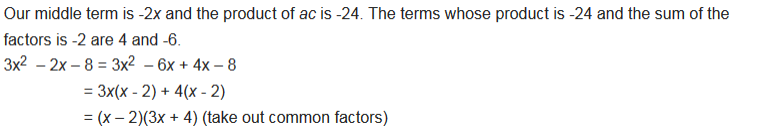Example 14
factorize x2 + 10x + 25 by splitting the middle term.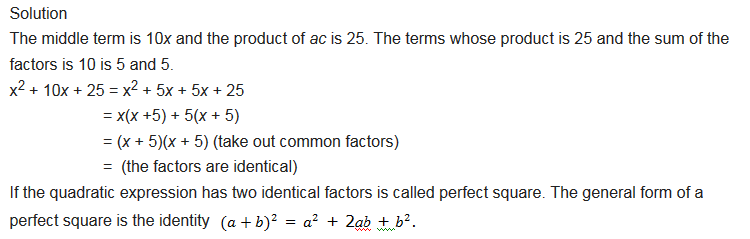Factorization by Inspection
Example 15
factorize x2 + 3x + 2 by inspection.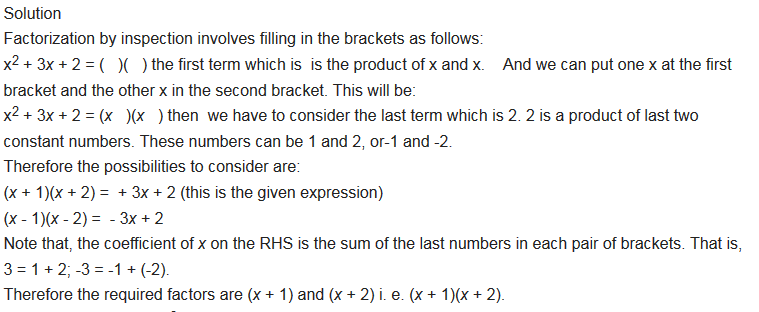Example 16

Exercise 1

1.Mwl.himbuka nyoni

Weka notes walau kwa pdf

2. Magnificent goods from you, man. I have understand
your stuff previous to and you are just extremely magnificent.
I actually like what you have acquired here, really
like what you’re saying and the way in which
you say it. You make it enjoyable and you still care for to keep it sensible.

I can not wait to read far more from you. This is really
a terrific website.

3. Nice post. I was checking constantly this blog and I’m impressed!

Very useful information particularly the last part 🙂 I care for such information a lot.
I was seeking this certain information for a long time.
Thank you and good luck.

4. This is really interesting, You’re an excessively professional blogger.
I’ve joined your feed and sit up for in the hunt for more of your